# Maximum Likelihood Estimation in the Ampadu-G Family of Distributions

Department of Biostatistics, USA

Received Date: March 29, 2019;  Published Date: April 26, 2019

#### Abstract

In this paper the maximum likelihood estimation for parameters in the Ampadu-G family of distributions  is discussed. When G is given by the normal distribution, we assess the performance of the estimation procedure via simulation study carried out in the software package R . As a further development we conjecture the methods of maximum product spacings and least squares are adequate for estimating the parameters in the Ampadu-G family of distributions.

Keywords: Ampadu-G; Normal distribution; Maximum likelihood estimation; Ordinary least squares estimation; Maximum product spacings estimation; Monte carlo simulation

#### Introduction and Preliminaries

At first, we recall the following

Definition 3.1:  Letλ > 0,ξ > 0 be a parameter vector all of whose entries are positive, and x ∈. A random variable X will be said to follow the Ampadu-G family of distributions if the CDF is given byand the PDF is given by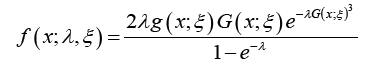where the baseline distribution has CDF G( x;ξ )and PDF g ( x;ξ )

#### The Quantile

Theorem 4.1. Let QG (•) = G−1 (•) denote the quantile of some base distribution with CDF G and PDF g,λ > 0, and 0 < u <1. The quantile function of the Ampadu-G family of distributions is given by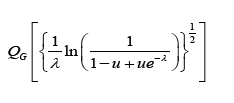Proof. Let 0 < u <1. Since QG (•) = G−1 (•) the quantile function can be obtained by solving the following equation for y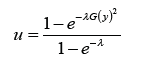#### Random Number Generation

If u U (0,1) , that is, u is a uniform random variable, then a random sample from the Ampadu-G family of distributions can be obtained viaWhere QG (•) = G−1 (•) denote the quantile of some base distribution with CDF G and PDF g, and λ > 0

#### Parameter Estimation

In this section, we obtain the maximum likelihood estimators (MLEs) for the parameters of the Ampadu-G family of distributions. For this, let X1,X2, · · · ,Xn be a random sample of size n from the Ampadu-G family of distributions. The likelihood function is given by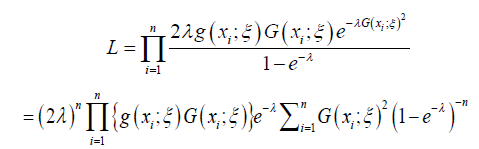From the above the log-likelihood function is given by

→ So we will have the final result:The MLE’s of ξ and λ can be obtained by maximizing the equation immediately above. The derivatives of the equation immediately above with respect to the unknown parameters are given as followsNow solving the system below forξ and λ gives the maximum likelihood estimators,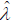and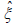of the unknown parameters:

So we have the following new model (ρ ,m,v) :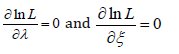#### Simulation Study

In this section, a Monte Carlo simulation study is carried out to assess the performance of the estimation method, when G is given by the normal distribution. First, we present the CDF and PDF of the Ampadu-Normal distribution.

Theorem 7.1. The CDF of the Ampadu-Normal family of distributions is given byBy differentiating the CDF in the previous theorem, we get the following

Theorem 7.2. The PDF of the Ampadu-Norma family of distribution is given byRemark 7.3. If a random variable W follows the Ampadu-Normal family of distributions, we write W ~ AN (λ,c, d ) (Figure 1 &2).Samples of sizes 200, 350, 500, and 700, are drawn from the Ampadu-Normal family of distributions. The samples have been drawn for (λ,c,d ) = (9.3, 3.8, 1.7) , and the maximum likelihood estimators for the parameters λ , c, and d are obtained. The procedure has been repeated 200 times and the mean and mean square error for the estimates are computed, and the results are summarized in Table 1 below.

Table 1: Result of Simulation Study.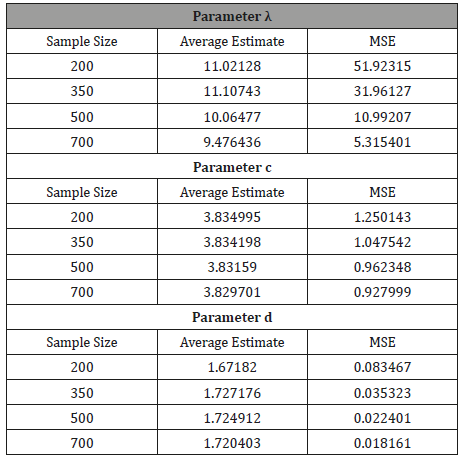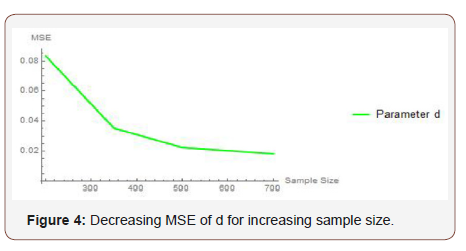From Table 1 above, we find that the simulated estimates are close to the true values of the parameters and hence the estimation method is adequate. We have also observed that the estimated mean square errors (MSEs) consistently decrease with increasing sample size as seen in the Figures below (Figure 3,4,5).

#### Concluding Remarks and Further Developments

In this paper we have shown that estimating the parameters in the Ampadu-Normal family of distributions by the method of maximum likelihood is adequate via a brief simulation study. As a further development we propose the following

Exercise 8.1. Conduct a simulation study to determine if the methods of maximum product spacings, and ordinary least squares are adequate in estimating the parameters in the Ampadu-Normal family of distributions.

Remark 8.2. The methods of maximum product spacings and ordinary least squares are discussed in .

None.

#### Ethics

No conflict of interest.

Article Details
Citation
Keywords
Scroll to
Scroll to Top